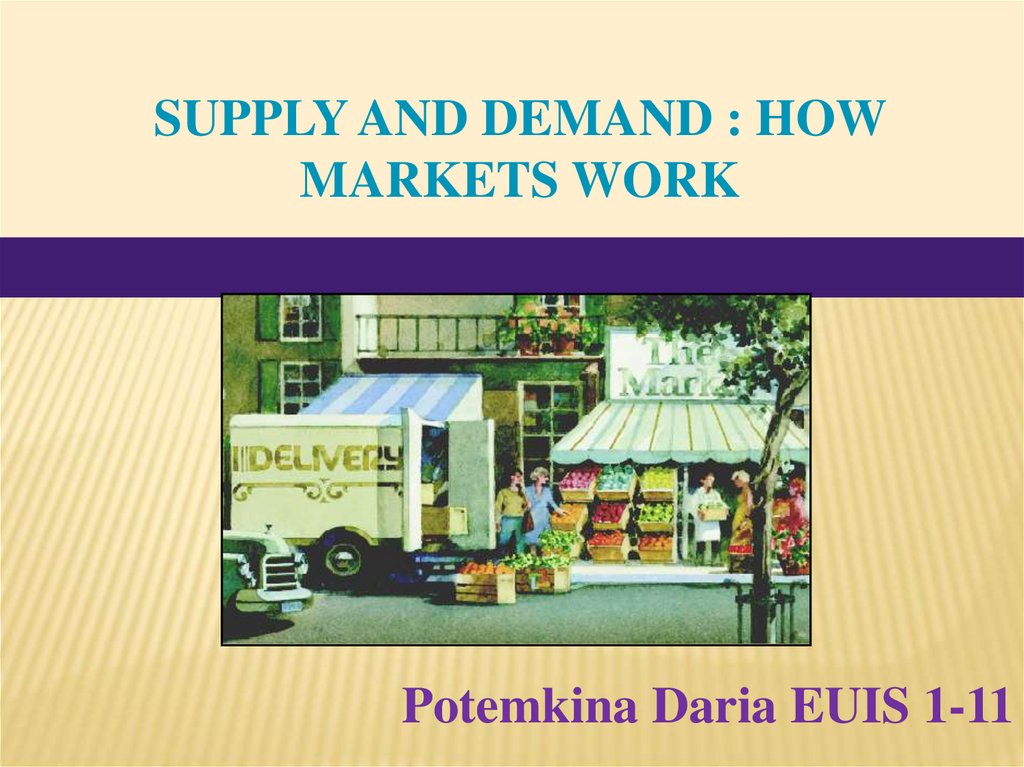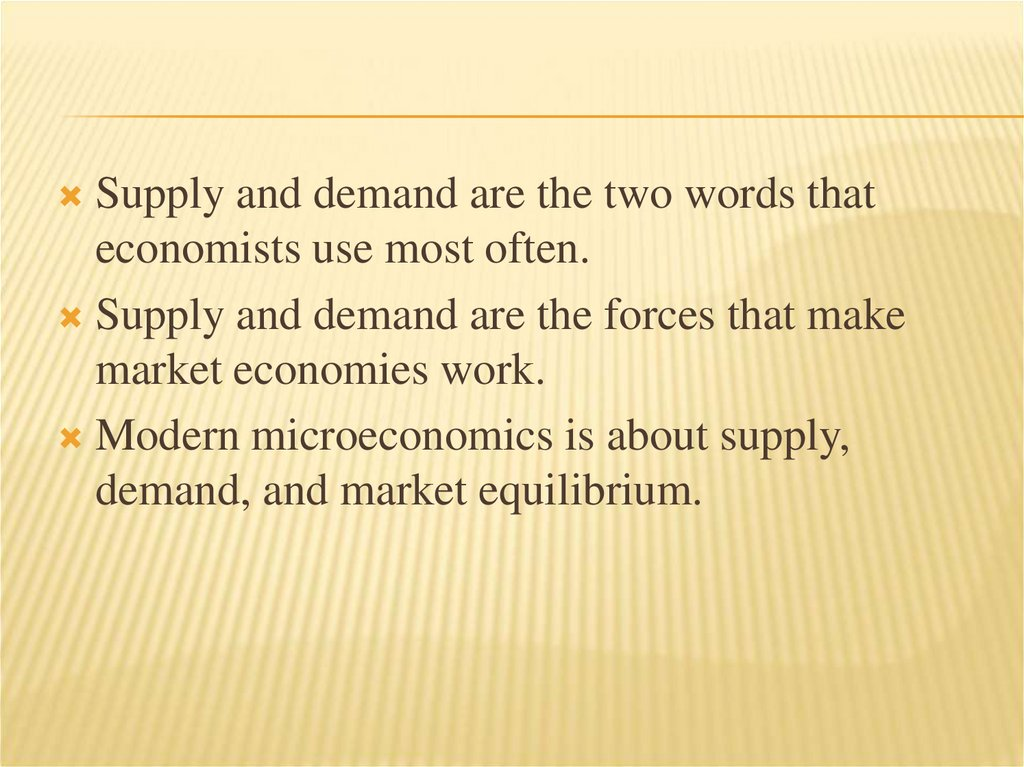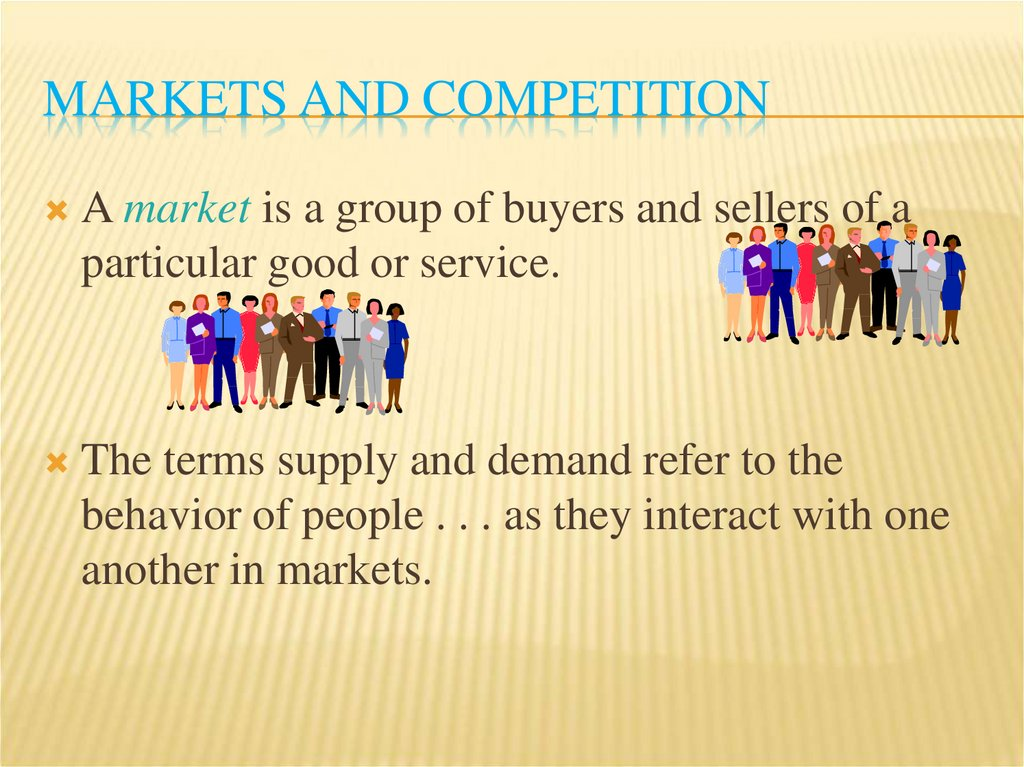# Supply and demand: how markets work

## 1.

SUPPLY AND DEMAND : HOW
MARKETS WORK
Potemkina Daria EUIS 1-11

## 2.

Supply and demand are the two words that
economists use most often.
Supply and demand are the forces that make
market economies work.
demand, and market equilibrium.

## 3. MARKETS AND COMPETITION

A market is a group of buyers and sellers of a
particular good or service.
The terms supply and demand refer to the
behavior of people . . . as they interact with one
another in markets.

## 4. MARKETS AND COMPETITION

Sellers determine supply

## 5. Competitive Markets

COMPETITIVE MARKETS
A competitive market is a market in which there
are many buyers and sellers so that each has a
negligible impact on the market price.

## 6. Competition: Perfect and Otherwise

COMPETITION: PERFECT AND OTHERWISE
Perfect Competition
Products
are the same
Numerous buyers and sellers so that each has no
influence over price
Buyers and Sellers are price takers
Monopoly
One
seller, and seller controls price

## 7. Competition: Perfect and Otherwise

COMPETITION: PERFECT AND OTHERWISE
Oligopoly
Few
sellers
Not always aggressive competition
Monopolistic Competition
Many
sellers
Slightly differentiated products
Each seller may set price for its own product

## 8. DEMAND

Quantity demanded is the amount of a good that
buyers are willing and able to purchase.
Law of Demand
The
law of demand states that, other things equal, the
quantity demanded of a good falls when the price of
the good rises.

## 9. The Demand Curve: The Relationship between Price and Quantity Demanded

THE DEMAND CURVE: THE RELATIONSHIP
BETWEEN PRICE AND QUANTITY DEMANDED
Demand Schedule
The
demand schedule is a table that shows the
relationship between the price of the good and the
quantity demanded.

## 10. The Demand Curve: The Relationship between Price and Quantity Demanded

THE DEMAND CURVE: THE RELATIONSHIP
BETWEEN PRICE AND QUANTITY DEMANDED
Demand Curve
The
demand curve is a graph of the relationship
between the price of a good and the quantity
demanded.

## 11. Market Demand versus Individual Demand

MARKET DEMAND VERSUS INDIVIDUAL
DEMAND
Market demand refers to the sum of all individual
demands for a particular good or service.
Graphically, individual demand curves are
summed horizontally to obtain the market
demand curve.

## 12. Shifts in the Demand Curve

SHIFTS IN THE DEMAND CURVE
Change in Quantity Demanded
Movement
along the demand curve.
Caused by a change in the price of the product.

## 13. Shifts in the Demand Curve

SHIFTS IN THE DEMAND CURVE
Consumer
income
Prices of related goods
Tastes
Expectations

## 14. Shifts in the Demand Curve

SHIFTS IN THE DEMAND CURVE
Change in Demand
A shift
in the demand curve, either to the left or right.
Caused by any change that alters the quantity
demanded at every price.

## 15. Shifts in the Demand Curve

SHIFTS IN THE DEMAND CURVE
Consumer Income
As
income increases the demand for a normal good
will increase.
As income increases the demand for an inferior good
will decrease.

## 16. Shifts in the Demand Curve

SHIFTS IN THE DEMAND CURVE
Prices of Related Goods
When
a fall in the price of one good reduces the
demand for another good, the two goods are called
substitutes.
When a fall in the price of one good increases the
demand for another good, the two goods are called
complements.

## 17. SUPPLY

Quantity supplied is the amount of a good that
sellers are willing and able to sell.
Law of Supply
The
law of supply states that, other things equal, the
quantity supplied of a good rises when the price of the
good rises.

## 18. The Supply Curve: The Relationship between Price and Quantity Supplied

THE SUPPLY CURVE: THE RELATIONSHIP
BETWEEN PRICE AND QUANTITY SUPPLIED
Supply Schedule
The supply schedule is a table that shows the
relationship between the price of the good and the
quantity supplied.
Supply Curve
The supply curve is the graph of the relationship
between the price of a good and the quantity supplied.

## 19. Market Supply versus Individual Supply

MARKET SUPPLY VERSUS INDIVIDUAL SUPPLY
Market supply refers to the sum of all individual
supplies for all sellers of a particular good or
service.
Graphically, individual supply curves are
summed horizontally to obtain the market supply
curve.

## 20. Shifts in the Supply Curve

SHIFTS IN THE SUPPLY CURVE
Input prices
Technology
Expectations
Number of sellers

## 21. Shifts in the Supply Curve

SHIFTS IN THE SUPPLY CURVE
Change in Quantity Supplied
Movement
along the supply curve.
Caused by a change in anything that alters the
quantity supplied at each price.
Change in Supply
A shift
in the supply curve, either to the left or right.
Caused by a change in a determinant other than price.

## 22. SUPPLY AND DEMAND TOGETHER

Equilibrium refers to a situation in which the
price has reached the level where quantity
supplied equals quantity demanded.

## 23. SUPPLY AND DEMAND TOGETHER

Equilibrium Price
The
price that balances quantity supplied and quantity
demanded.
On a graph, it is the price at which the supply and
demand curves intersect.
Equilibrium Quantity
The
quantity supplied and the quantity demanded at
the equilibrium price.
On a graph it is the quantity at which the supply and
demand curves intersect.

## 24. Equilibrium

EQUILIBRIUM
Surplus
When
price > equilibrium price, then quantity
supplied > quantity demanded.
There
is excess supply or a surplus.
Suppliers will lower the price to increase sales, thereby
moving toward equilibrium.

## 25. Equilibrium

EQUILIBRIUM
Shortage
When
price < equilibrium price, then quantity
demanded > the quantity supplied.
There is excess demand or a shortage.
Suppliers will raise the price due to too many
buyers chasing too few goods, thereby moving
toward equilibrium.

## 26. Equilibrium

EQUILIBRIUM
Law of supply and demand
The
claim that the price of any good adjusts to bring
the quantity supplied and the quantity demanded for
that good into balance.

## 27. Three Steps to Analyzing Changes in Equilibrium

THREE STEPS TO ANALYZING CHANGES IN
EQUILIBRIUM
Decide whether the event shifts the supply or
demand curve (or both).
Decide whether the curve(s) shift(s) to the left or
to the right.
Use the supply-and-demand diagram to see how
the shift affects equilibrium price and quantity.

## 28. Three Steps to Analyzing Changes in Equilibrium

THREE STEPS TO ANALYZING CHANGES IN
EQUILIBRIUM
Shifts in Curves versus Movements along Curves
A shift
in the supply curve is called a change in
supply.
A movement along a fixed supply curve is called a
change in quantity supplied.
A shift in the demand curve is called a change in
demand.
A movement along a fixed demand curve is called a
change in quantity demanded.

## 29. Summary

SUMMARY
Economists use the model of supply and demand
to analyze competitive markets.
In a competitive market, there are many buyers
and sellers, each of whom has little or no
influence on the market price.

## 30. Summary

SUMMARY
The demand curve shows how the quantity of a good
depends upon the price.
According to the law of demand, as the price of a good
falls, the quantity demanded rises. Therefore, the demand
curve slopes downward.
In addition to price, other determinants of how much
consumers want to buy include income, the prices of
complements and substitutes, tastes, expectations, and the
If one of these factors changes, the demand curve shifts.

## 31. Summary

SUMMARY
The supply curve shows how the quantity of a
good supplied depends upon the price.
According
to the law of supply, as the price of a good
rises, the quantity supplied rises. Therefore, the
supply curve slopes upward.
In addition to price, other determinants of how much
producers want to sell include input prices,
technology, expectations, and the number of sellers.
If one of these factors changes, the supply curve
shifts.

## 32. Summary

SUMMARY
Market equilibrium is determined by the
intersection of the supply and demand curves.
At the equilibrium price, the quantity demanded
equals the quantity supplied.
The behavior of buyers and sellers naturally
drives markets toward their equilibrium.

## 33. Summary

SUMMARY
To analyze how any event influences a market,
we use the supply-and-demand diagram to
examine how the even affects the equilibrium
price and quantity.
In market economies, prices are the signals that
guide economic decisions and thereby allocate
resources.